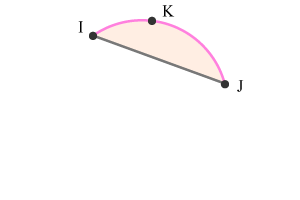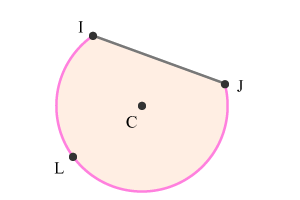# Circular segment

## Definition

A part of the circle enclosed by an arc and a chord is called a segment of the circle.

A chord is a line segment in the circle and it is formed by joining any two points on the circumference of the circle. The endpoints of the chord are points on circumference and they are also endpoints to two arcs. In other words, the points are common endpoints to the two arcs and also to the chord. The area enclosed by each arc with the chord is part of the circle and it is called as segment of the circle.

## Types

Any two points on the circumference of the circle form two types of segments by a chord and two arcs.

1. Minor segment
2. Major segment

Consider two points $I$ and $J$ on the circumference of a circle. Join $I$ and $J$ to form a line segment $\stackrel{‾}{IJ}$. The points $I$ and $J$ are endpoints to the line segment $\stackrel{‾}{IJ}$ and also formed two arcs by dividing the circumference into two parts.

1.

### Minor SegmentMinor segment of a Circle

Consider a point $K$ on the circumference of the circle. The points $I$ and $J$ with point $K$ formed an arc $IKJ$ geometrically.

The chord $\stackrel{‾}{IJ}$ with arc $IKJ$ represents a part of the circle and it is known as a segment. If the area of the segment is minimum when compared to other part of the circle, the segment is called as minor segment.

In this example, the segment formed by the arc $IKJ$ and chord $\stackrel{‾}{IJ}$ is a minor segment due to the minor area of part of the circle.

2.

### Major SegmentMajor segment of a Circle

Consider a point $L$ on the circumference of the circle. The points $I$ and $J$ with point $L$ formed an arc $ILJ$ geometrically.

The arc $ILJ$ and chord $\stackrel{‾}{IJ}$ represents a part of the circle and it is called as a segment. However, if the area of the segment is maximum than the area of other part of the circle, the segment is called as major segment.

In this example, the segment that is formed by the chord $\stackrel{‾}{IJ}$ and arc $ILJ$ is an example for major segment because of the area of part of the circle.

If in case, the chord is diameter of the circle, the two segments are minor as well as major segments of the circle. Each segment of the circle is called as semicircle in this special case.

Latest Math Topics
Jun 26, 2023
Jun 23, 2023

###### Math Questions

The math problems with solutions to learn how to solve a problem.

Learn solutions

Practice now

###### Math Videos

The math videos tutorials with visual graphics to learn every concept.

Watch now

###### Subscribe us

Get the latest math updates from the Math Doubts by subscribing us.